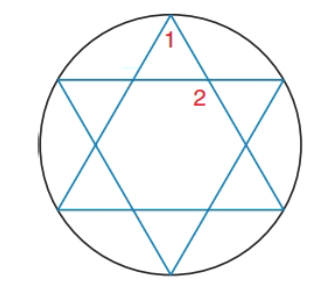Chapter 6.2, Problem 30EElementary Geometry For College St...

7th Edition
Alexander + 2 others
ISBN: 9781337614085

Solutions

Chapter
SectionElementary Geometry For College St...

7th Edition
Alexander + 2 others
ISBN: 9781337614085
Textbook Problem

For the six-pointed star ( a regular hexagram) inscribed in the circle, find the measures of ∠ 1   and ∠ 2 .To determine

To find:

The measure of 1and2.

Explanation

Given:

Calculation:

If 1 has the vertex on the circle:

mA=m1=mCDE2=mCD+mDE2=2mCD2=mCD=360°6=60°

If 2 has the vertex inside the circle.

Still sussing out bartleby?

Check out a sample textbook solution.

See a sample solution

The Solution to Your Study Problems

Bartleby provides explanations to thousands of textbook problems written by our experts, many with advanced degrees!

Get Started

Graph the following functions. 5.

Mathematical Applications for the Management, Life, and Social Sciences

Finding the Domain In Exercises 41-46, find the domain of the function. f(x)=12ex

Calculus: Early Transcendental Functions (MindTap Course List)

limx1x3x21x = _____. a) 1 b) 1 c) 0 d) does not exist

Study Guide for Stewart's Single Variable Calculus: Early Transcendentals, 8th